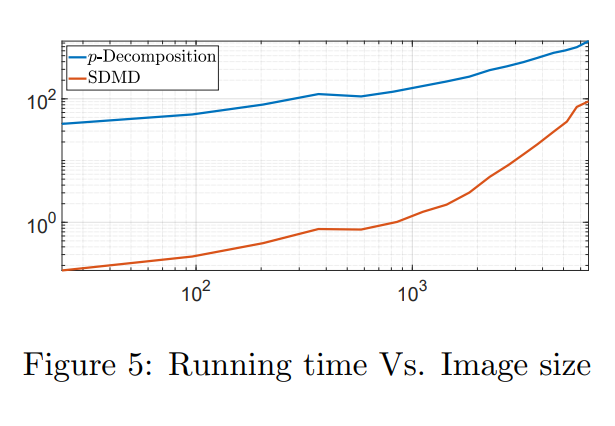# Modes of Homogeneous Gradient Flows

Accepted to SIAM J. on Imaging Sciences, 2021

Ido Cohen, Omri Azencot, Pavel Lifshitz, Guy Gilboa, arXiv, July 2020

Finding latent structures in data is drawing increasing attention in broad and diverse fields such as fluid dynamics, signal processing, and machine learning. In this work, we formulate Dynamic Mode Decomposition (DMD) for two types of dynamical system. The first, a system which is derived by a $\gamma$-homogeneous operator ($\gamma\neq 1$).
The second, a system which can be represented as a symmetric operator.

Regarding to the first type, dynamical systems, derived by $\gamma$-homogeneous operators $\gamma\in[0,1)$, reach the steady state in finite time. This inherently contradicts the DMD model, which can be seen as an exponential data fitting algorithm. Therefore, the induced DMD operator leads to artifacts in the decomposition. We show certain cases where the DMD does not even exist. For homogeneous systems ($\gamma\neq 1$), we suggest a time rescaling that solves this conflict and show that DMD can perfectly restore the dynamics even for nonlinear flows. For dynamics which derived by a symmetric operator, we expect the eigenvalues of the DMD to be real. This requirement is embeded in a variant of the DMD algorithm, termed as Symmetric DMD  (SDMD).

With these adaptations, we formulate a closed form solution of DMD for dynamics $u_t = P(u)$, $u(t=0)=u_0$, where $P$ is a nonlinear $\gamma$-homogeneous operator, when the initial condition $u_0$ admits the nonlinear eigenvalue problem $P(u_0)=\lambda u_0$ ($u_0$ is a nonlinear eigenfunction, with respect to the operator $P$). We show experimentally that, for such systems, for any initial condition, SDMD achieves lower mean square error for the spectrum estimation. Finally, we formulate a discrete decomposition, related to nonlinear eigenfunctions of $\gamma$-homogeneous operator.